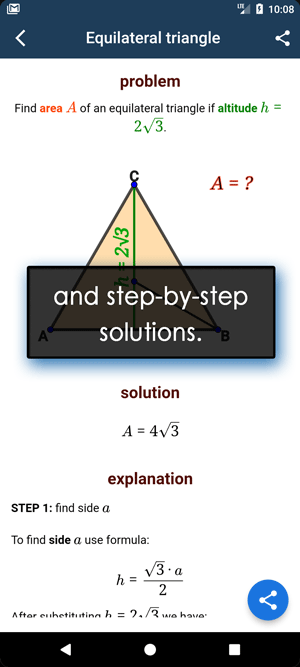Math Calculators, Lessons and Formulas

It is time to solve your math problem

mathportal.org

# GCD calculator

This calculator computes Greatest Common Divisor (GCD) of two or more numbers using four different methods.

GCD Calculator
four calculation methods with steps for each of them
show help ↓↓ examples ↓↓
Find GCD using prime factorization method
Find GCD using repeated division method
Euclidean Algorithm (works for two numbers)
Listing out the factorsworking...
examples
example 1:ex 1:
What is the Greatest Common Divisor (GCD) of 104 and 64?
example 2:ex 2:
Find GCD of 96, 144 and 192 using a repeated division.
example 3:ex 3:
Find GCD of 54 and 60 using an Euclidean Algorithm.
example 4:ex 4:
Find GCD of 72 and 54 by listing out the factors.

## GCD methods

This calculator uses four methods to find GCD. We will show them using few examples.

### Method 1 : Find GCD using prime factorization method

Example: find GCD of 36 and 48

Step 1: find prime factorization of each number:

42 = 2 * 3 * 7

70 = 2 * 5 * 7

Step 2: circle out all common factors:

42 = * 3 *

70 = * 5 *

We see that the GCD is * = 14

### Method 2 : Find GCD using a repeated division

Example: find GCD of 84 and 140.

Step 1: Place the numbers inside division bar:

 84 140

Step 2: Divide both numbers by 2:

 2 84 140 42 70

Step 3: Continue to divide until the numbers do not have a common factor.

 ② 84 140 ② 42 70 ⑦ 21 35 3 7

Step 4: The GCD of 84 and 140 is: * * = 28

### Method 3 : Euclidean algorithm

Example: Find GCD of 52 and 36, using Euclidean algorithm.

Solution: Divide 52 by 36 and get the remainder, then divide 36 with the remainder from previous step. When the remainder is zero the GCD is the last divisor.

 52 : 36 = 1 remainder (16) 36 : 16 = 1 remainder (4) 16 : ④ = 4 remainder (0)

We conclude that the GCD = 4.

### Method 4 : Listing out the factors

Example: find GCD of 45 and 54 by listing out the factors.

Step 1: Find all divisors of the given numbers:

The divisors of 45 are 1, 3, 5, , 15 and 45

The divisors of 54 are 1, 2, 3, 6, 18, 27 and 54

Step 2: The greatest divisor =

Search our database of more than 200 calculators